# Radiant Flux and Luminous Flux: What are they?

The electromagnetic radiation with the wavelength from 100 nm and 1 mm is referred as the optical range of radiation. Inside this range, the electromagnetic radiation with wavelength 380 nm to 780 nm is called light and this range is the visible range of wavelength. The optical radiation with wavelengths shorter than 380 nm is called ultraviolet radiation, similarly, the optical radiation with wavelength more than 780 nm is called infrared radiation.

Another point to be noted is that, most of the power from a radiation source is radiated within optical range of wavelength. Although as per “theory of black body radiation” power is radiated at all wavelengths (from zero to infinity) but it is found that the amount of power radiated for the wavelengths other than that in optical range is negligible. As the entire amount of power is radiated in the optical range of wavelengths, it can be said that a 100 W incandescent lamp would radiate 100 W power.

Radiant flux (also known as radiant power) is the radiant energy emitted, reflected, transmitted or received, per unit time. The SI unit of radiant flux is the watt (W), that is the joule per second (J/s) in SI base units.

From any radiation source, the radiated energy per unit time is referred as the radiant flux. Suppose this radiated energy is denoted as Q joule then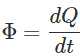Where, Φ is the radiated energy per second and its unit is watt. That means radiant flux is nothing but radiated power.
Although it has already been considered that entire power or flux is radiated within optical range of wavelength but the radiant flux is not equal for all the wavelength. It varies with wavelength. Let us consider Φλ(λ) is radiant flux at wavelength λ.

Hence for entire optical range of wavelength the radiant flux will be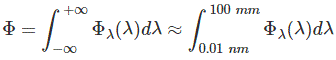Now if this radiation is within human visual sensation then this radiant flux is called luminous flux.

## What is Luminous Flux?

Luminous flux has a unit i.e. Lumen (lm) instead of watt. The visible range of wavelengths is quite narrow compared to the optical range and sensitivity of human eyes varies considerably with the wavelength within this visible range. The sensitivity of human eyes to the different wavelengths of light can be represented by spectral sensitivity function or luminous efficacy function Vλ.

This is actually proportional to relative spectral sensitivity function V(λ). The sensitivity of human eyes in photopic vision is maximum at wavelength 555 nm. The relative spectral sensitivity at that wavelength is taken as 1. The luminous efficacy at the wavelength of 555 nm is 683 lumens/watt. Hence, luminous efficacy at any wavelength within visible range can be found from the equation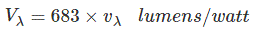Where, vλ is relative spectral sensitivity at wavelength λ that means it is the value of relative spectral sensitivity function V(λ) at wavelength λ.

Here, Vλ lumens is correlated to one-watt radiant flux at wavelength λ so at Φλ watts radiant flux, there will be ΦλVλ lumens. This quantity is defined as luminous flux at wavelength λ. For entire visible range of wavelength, the luminous flux will be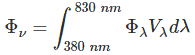This is the conversion equation that is used to quantify any photometric quantity from the radiometric quantity and we use the equation when we try to correlate the radiant flux and the luminous flux.
We can represent the spectral efficacy in the graph of the perceived optical stimulus vs. the incident radiant power as a function of wavelength. The human eye is almost 2.5 times as sensitive at the wavelength of maximum sensitivity when it is dark.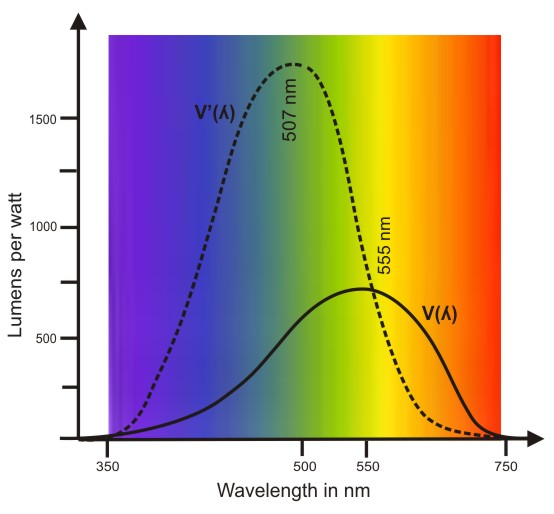Want To Learn Faster? 🎓
Get electrical articles delivered to your inbox every week.
No credit card required—it’s 100% free.